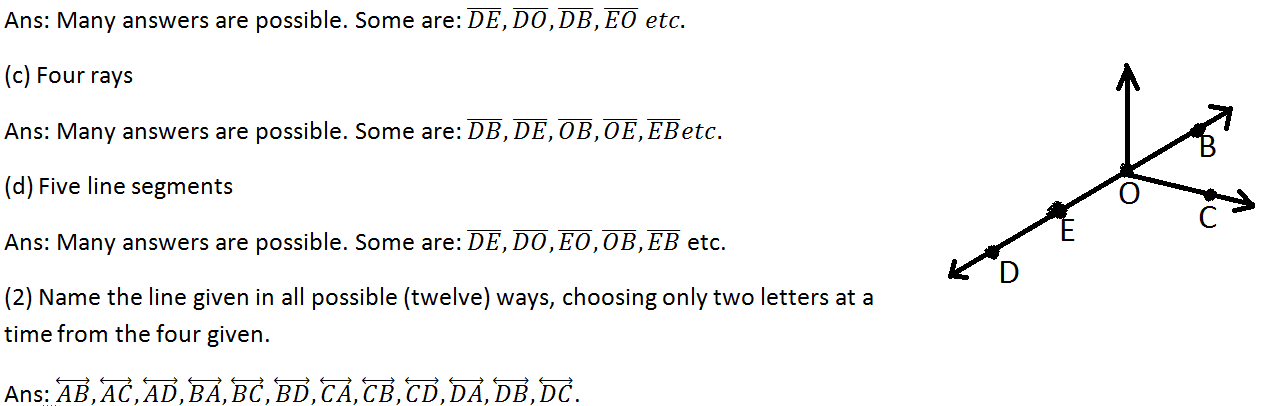# NCERT Class 6 Mathematics First Chapter Knowing Our Numbers Exercise 1.2 Solution

## EXERCISE 1.2

(1) A book exhibition was held for four days in a school. The number of tickets sold at the counter on the first, second, third and final day was respectively 1094, 1812, 2050 and 2751. Find the total number of tickets sold on all the four days.

Solution: The total number of tickets sold = (1094 + 1812 + 2050 + 2751) = 7077

Therefore, all the four days was sold 7077 tickets.

(2) Shekhar is a famous cricket player. He has so far scored 6980 runs in test matches. He wishes to complete 10,000 runs. How many more runs does he need?

Solution: (10,000 – 6980) = 3020

Therefore, Shekhar needs 3020 runs.

(3) In an election, the successful candidate registered 5,77,500 votes and his nearest rival secured 3,48,700 votes. By what margin did the successful candidate win the election?

Solution: (5,77,500 – 3,48,700) = 228800

Hence, the successful candidate win the election by 228800 votes.

(4) Kirti bookstore sold books worth Rs 2,85,891 in the first week of June and books worth Rs 4,00,768 in the second week of the month. How much was the sale for the two weeks together? In which week was the sale greater and by how much?

Solution: The bookstore sold for the two weeks together = Rs (2,85,891 + 4,00,768) = Rs 686659

And (400768 – 285891) = 114857 rupees

Hence, Second week was the sale greater by Rs 1,14,857.

(5) Find the difference between the greatest and the least 5-digit number that can be written using the digits 6, 2, 7, 4, 3 each only once.

Solution: The greatest number is 76432 and the least number is 23467.

Therefore, the difference between the two number = (76432 – 23467) = 52965.

(6) A machine, on an average, manufactures 2,825 screws a day. How many screws did it produce in the month of January 2006?

Solution: In January, 2006 the machine manufactures = (2825 × 31) = 87575 screws.(7) A merchant had Rs 78,592 with her. She placed an order for purchasing 40 radio sets at Rs 1200 each. How much money will remain with her after the purchase?

Solution: The merchant spend money for 40 radio sets = Rs (1200 × 40) =Rs 4800

Therefore, (78592 – 48000) rupees = 30592 rupees will remain with her after the purchase.

(8) A student multiplied 7236 by 65 instead of multiplying by 56. By how much was his answer greater than the correct answer?

Solution: (7236 × 65) = 470340 And (7236 × 56) = 405216

Therefore, the greater answer is 470340 by (470340 – 405216) = 65124.

In case you are missed :- Previous Chapter Exercise Solution

(9) To stitch a shirt, 2 m 15 cm cloth is needed. Out of 40 m cloth, how many shirts can be stitched and how much cloth will remain?

Solution: Here, 40 m = 4000 cm and 2 m 15 cm = 215 cmHence, 18 shirts can be stitched and 130 cm / 1 m 30 cm cloth will remain.

(10) Medicine is packed in boxes, each weighing 4 kg 500g. How many such boxes can be loaded in a van which cannot carry beyond 800 kg?

Solution: Here, 800 kg = 800, 000 g and 4 kg 500g = 4500 g

Hence, 177 boxes can be loaded in the van.(11) The distance between the school and a student’s house is 1 km 875 m. Every day she walks both ways. Find the total distance covered by her in six days.

Solution: Here 1 km 875 m = 1875 m

Therefore, the student one day walks of the ways = (1875 × 2) m = 3650 m

Hence, the total distance covered by her in six days is 21 km 900 m.(12) A vessel has 4 litres and 500 ml of curd. In how many glasses, each of 25 ml capacity, can it be filled?

Solution: Here, 4 litres and 500 ml = 4500 ml

Therefore, 180 glasses can the vessel filled.In case you are missed :- Next Chapter Exercise Solution

Updated: April 14, 2023 — 1:08 pm